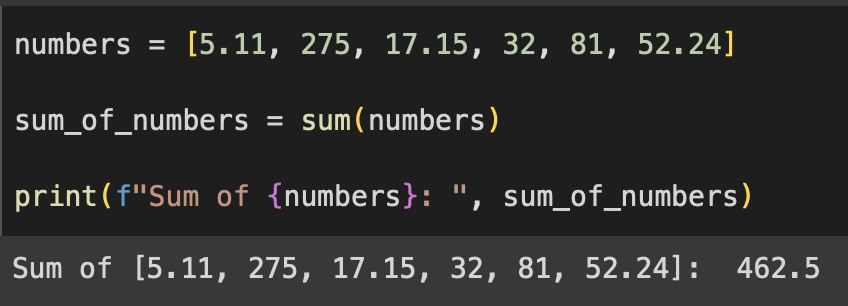# Python: Calculate Sum of numbers as elements of a List

We can make use of the the built-in sum() function. Let's take a look at a few examples.

## Example 1: Calculate the sum of a list of integers

``````int_numbers = [11, 5, 2, 17, 32, 81, 52]

sum_of_int_list = sum(int_numbers)

print(f"Sum of {int_numbers}: ", sum_of_int_list)``````
Output:
``Sum of [11, 5, 2, 17, 32, 81, 52]:  200``

## Example 2: Calculate the sum of a list of floats

``````float_numbers = [11.2, 5.11, 2.75, 17.56, 32.43, 81.45, 52.24]

sum_of_float_list = sum(float_numbers)

print(f"Sum of {float_numbers}: ", sum_of_float_list)``````
``Sum of [11.2, 5.11, 2.75, 17.56, 32.43, 81.45, 52.24]:  202.74``

## Example 3: Sum of random numbers-

### Facing issues? Have Questions? Post them here! I am happy to answer!Author Info:

Rakesh is a seasoned developer with over 10 years of experience in web and app development, and a deep knowledge of operating systems. Author of insightful How-To articles for Code2care.